### Electric Circuit and Electron Device–Important 6 Marks Questions with Answers

Anna University

Electric Circuit and Electron Device

Subject Code : EC2151

Subject Name : Electric Circuit and Electron Device

Year : 1st yr

Semester : 2nd Sem

Department : CSE & ECE

Important 6 MARKS Questions with Answers

1. A 3 φ 4 pole 50 hz induction motor runs at 1460 r.p.m. find its % of slip.

Solution

N s = 120f/p

= 120*50/4

= 1500r.p.m. Running speed of motor

n= 1460r.p.m. Slip S=( N s–N)/ N s*100

=(1500-1460) x 100 / 1500

= 2.667%

2. Explain the working principle of Transformer.

A Transformer is a device that transfers electrical energy from one circuit to another by electromagnetic induction (transformer action). The electrical energy is always transferred without a change in frequency, but may involve changes in magnitudes of voltage and current. Because a transformer works on the principle of electromagnetic induction, it must be used with an input source voltage that varies in amplitude. There are many types of power that fit this description; for ease of explanation and understanding, transformer action will be explained using an ac voltage as the input source.

The amount of power used by the load of an electrical circuit is equal to the current in the load times the voltage across the load, or P = EI. If, for example, the load in an electrical circuit requires an input of 2 amperes at 10 volts (20 watts) and the source is capable of delivering only 1 ampere at 20 volts, the circuit could not normally be used with this particular source. However, if a transformer is connected between the source and the load, the voltage can be decreased (stepped down) to 10 volts and the current increased (stepped up) to 2 amperes. Notice in the above case that the power remains the same. That is, 20 volts times 1 ampere equals the same power as 10 volts times 2 amperes.

A Transformer consists of the following parts

A primary coil or winding.

A secondary coil or winding.

A core that supports the coils or windings.

The primary winding is connected to a 50 hertz ac voltage source. The magnetic field (flux) builds up (expands) and collapses (contracts) about the primary winding. The expanding and contracting magnetic field around the primary winding cuts the secondary winding and induces an alternating voltage into the winding. This voltage causes alternating current to flow through the load. The voltage may be stepped up or down depending on the design of the primary and secondary windings.

3. Calculate the amount of resistance (R) in a circuit, given values of voltage (E) and current (I):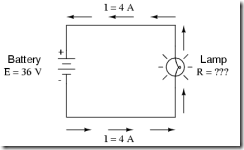the amount of resistance (R) offered by the lamp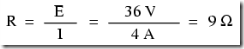4. calculate the amount of voltage supplied by a battery, given values of

current (I) and resistance (R):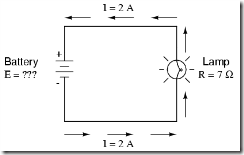the amount of voltage provided by the battery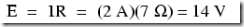5. Calculate the electric power in the given circuit and discuss the effect of increasing the battery voltage.

The formula for determining the power in an electric circuit: by multiplying the voltage in "volts" by the current in "amps" we arrive at an answer in "watts." Let's apply this to the given circuit.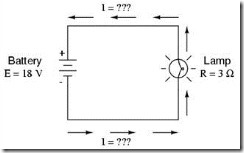In the above circuit, we know we have a battery voltage of 18 volts and a

lamp resistance of 3 Ω. Using Ohm's Law to determine current, we get: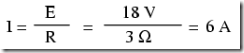Now that we know the current, we can take that value and multiply it by

the voltage to determine power: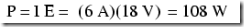6. What is meant by DEFLECTING TORQUE ?

The deflecting torque is produced by making use of one of the magnetic, chemical, electrostatic and electromagnetic induction effects of current or voltage and causes the moving system of the instrument to move from its zero position when the instrument is connected in an electrical circuit to measure the electrical quantity. The method of producing this torque depend upon the type of instrument. In attracting the type of instrument, this torque to equal to

Td = 1/2 I2 dL/dθ

Whereas in Pmmc instruments

Td = Bilur

Where B - magnetic density

i - current flowing l - length of coil

u - number of turn r - radius of coil

7. Find the voltage across each resistors in the following circuit.

8. The effective resistance of two resistors connected in series is 100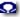.

When connected in parallel, then effective value in 24 ohm’s. Determine the value of two resistors

R1R2/R1+R2 = 24

R1R2/100 = 24

R1R2 =2400

R1 (100-R1) = 2400

100 R1-R1^2-2400 = 0

R1^2-100 R1 + 2400 = 0 (R1-60)(R1-40) = 0

There Fore R1 = 60; R1 = 40

9. Find the Req between two points A & B.

1/Req = ½+1/3+1/3 = 1.17 (Req = 1/1.17= 0.8547)

1/Req = 2+.85+4

10. Explain about Krichoffs voltage and current laws.

Kirchhoff’s Current Law

The sum of current flowing towards a function is equal to the current

flowing away from it.

Consider a function formed by 6 conductors. The current in these conductors are i1, i2, .i6.Some of these currents are flowing towards a 8 other’s away from A

According to Kirchhoff’s Law, i1+i4+i5+i6 = i2+i3

(Flowing towards) (Flowing away from A)

Kirchhoff’s Voltage Law (II Law)

In a closed circuit, the sum of the potential drops is equal to the sum of the potential resistance

ABCDA forms a closed circuit.

From A -> B, We have a potential drop of IR1. From D -> A, We have a potential drop of V. Sum of potential drops = IR1+IR2+IR3

Potential rise from D -> A =V IR1+IR2+IR3 = V

11. Explain the principle of operation of DC Motor.

When a current passes through a conductor, lines of magnetic force (flux) are generated around the conductor. The direction of the flux is dependent on the direction of the current flow. In terms of conventional current flow (positive to negative) then, using your right hand point your thumb in the direction of the current flow and your fingers will wrap around the conductor in the same direction of the flux lines.

On the side of the conductor where the lines of flux oppose each other, the magnetic field will be made weaker. On the side of the conductor where the lines of flux are not opposing each other, the magnetic field will be made stronger. Because of the strong field on one side of the conductor and a weak field or, the other side, the conductor will be pushed into the weaker field.

The armature is connected to the commutator which rides along the brushes which are connected to a DC power source. The current from the DC power source flows from the positive lead, through the brush labeled A1 through one commutator section, through the armature coil, through the other commutator section, through the brush labeled A2 and back to the negative lead.This current will generate lines of flux around the armature and affect the lines of flux in the air gap. On the side of thecoil where the lines of flux

oppose each other, the magnetic field will be made weaker. On the side of the coil where the lines of flux are riot opposing each other, the magnetic field is made stronger. Because of the strong field on one side of the coil and the weak field on the other side, the coil will be pushed into the weaker field and, because the armature coil is free to rotate, it will rotate.

The torque available at the motor shaft (turning effort) is determined by the magnetic force (flux) acting on the armature coil and the distance from the renter of rotation that force is. The flux is determined by the current flowing through the armature coil and strength of the field magnets.

12. Explain the working principle of three phase induction motor.

In three phase induction motor, the magnetic field generated by the stator rotates in the ac case. Three electrical phases are introduced through terminals, each phase energizing an individual field pole. When each phase reaches its maximum current, the magnetic field at that pole reaches a maximum value. As the current decreases, so does the magnetic field. Since each phase reaches its maximum at a different time within a cycle of the current, that field pole whose magnetic field is largest is constantly changing between the three poles, with the effect that the magnetic field seen by the rotor is rotating. The speed of rotation of the magnetic field, known as the synchronous speed, depends on the frequency of the power supply and the number of poles produced by the stator winding. For a standard 60 Hz supply, as used in the United States, the maximum synchronous speed is 3,600 rpm.

In the three phase induction motor, the windings on the rotor are not connected to a power supply, but are essentially short circuits. The most common type of rotor winding, the squirrel cage winding, bears a strong resemblance to the running wheel used in cages for pet gerbils. When the motor is initially switched on and the rotor is stationary, the rotor conductors experience a changing magnetic field sweeping by at the synchronous speed.

From Faraday's law, this situation results in the induction of currents round the rotor windings; the magnitude of this current depends on the impedance of the rotor windings. Since the conditions for motor action are now fulfilled, that is, current carrying conductors are found in a magnetic field, the rotor experiences a torque and starts to turn. The rotor can never rotate at the synchronous speed because there would be no relative motion between the magnetic field and the rotor windings and no current could be induced. The induction motor has a high starting torque.

13. Explain the working principle of single phase induction motor.

Single phase induction motor has only one stator winding (main winding) and operates with a single-phase power supply. In all single-phase induction motors, the rotor is the squirrel cage type. The single-phase induction motor is not self-starting. When the motor is connected to a single-phase power supply, the main winding carries an alternating current. This current produces a pulsating magnetic field. Due to induction, the rotor is energized. As the main magnetic field is pulsating, the torque necessary for the motor rotation is not generated. This will cause the rotor to vibrate, but not to rotate. Hence, the single phase induction motor is required to have a starting mechanism that can provide the starting kick for the motor to rotate.

The starting mechanism of the single-phase induction motor is mainly an additional stator winding (start/ auxiliary winding) as shown in Figure. The start winding can have a series capacitor and/or a centrifugal switch. When the supply voltage is applied, current in the main winding lags the supply voltage due to the main winding impedance. At the same time, current in the start winding leads/lags the supply voltage depending on the starting mechanism impedance. Interaction between magnetic fields generated by the main winding and the starting mechanism generates a resultant magnetic field rotating in one direction. The motor starts rotating in the direction of the resultant magnetic field. Once the motor reaches about 75% of its rated speed, a centrifugal switch disconnects the start winding. From this point on, the single-phase motor can maintain sufficient torque to operate on its own.

14. Explain the working principle of single phase Energy Meter.

An electric meter or energy meter is a device that measures the amount of electrical energy supplied to or produced by a residence, business or machine.

The most common type is a kilowatt hour meter. When used in electricity retailing, the utilities record the values measured by these meters to generate an invoice for the electricity. They may also record other variables including the time when the electricity was used.

Modern electricity meters operate by continuously measuring the instantaneous voltage (volts) and current (amperes) and finding the product of these to give instantaneous electrical power (watts) which is then integrated against time to give energy used (joules, kilowatt-hours etc). The meters fall into two basic categories, electromechanical and electronic.

The energy meter operates by counting the revolutions of an aluminium disc which is made to rotate at a speed proportional to the power. The number of revolutions is thus proportional to the energy usage. It consumes a small amount of power, typically around 2 watts.

The metallic disc is acted upon by two coils. One coil is connected in such a way that it produces a magnetic flux in proportion to the voltage and the other produces a magnetic flux in proportion to the current. The field of the voltage coil is delayed by 90 degrees using a lag coil. This produces eddy currents in the disc and the effect is such that a force is exerted on the disc in proportion to the product of the instantaneous current and voltage. A permanent magnet exerts an opposing force proportional to the speed of rotation of the disc - this act as a brake which causes the disc to stop spinning when power stops being drawn rather than allowing it to spin faster and faster. This causes the disc to rotate at a speed proportional to the power being used.

The type of meter described above is used on a single-phase AC supply. Different phase configurations use additional voltage and current coils.

The aluminium disc is supported by a spindle which has a worm gear which drives the register. The register is a series of dials which record the amount of energy used. The dials may be of the cyclometer type, an odometer-like display that is easy to read where for each dial a single digit is shown through a window in the face of the meter, or of the pointer type where a pointer indicates each digit. It should be noted that with the dial pointer type, adjacent pointers generally rotate in opposite directions due to the gearing mechanism.